# Digital Design Sequential Logic Design Chapter 3 Sequential

• Slides: 49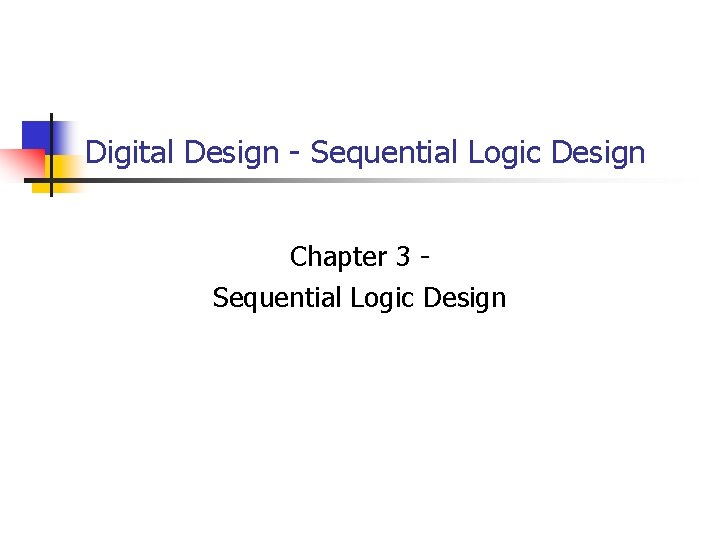Digital Design - Sequential Logic Design Chapter 3 Sequential Logic Design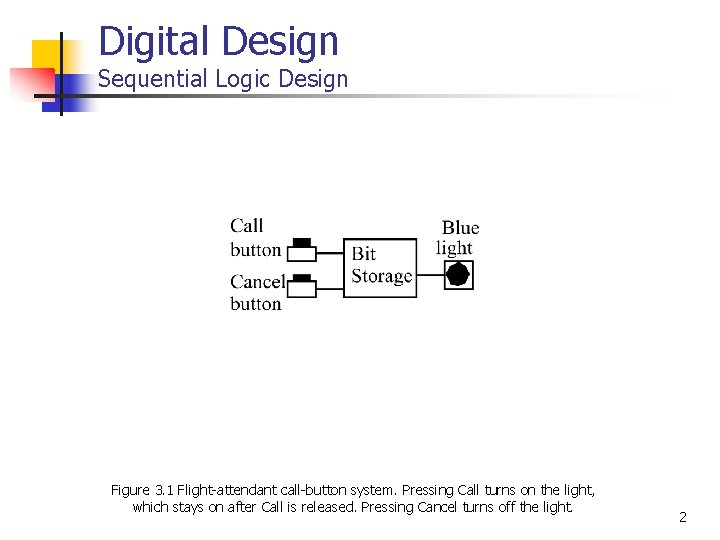Digital Design Sequential Logic Design Figure 3. 1 Flight-attendant call-button system. Pressing Call turns on the light, which stays on after Call is released. Pressing Cancel turns off the light. 2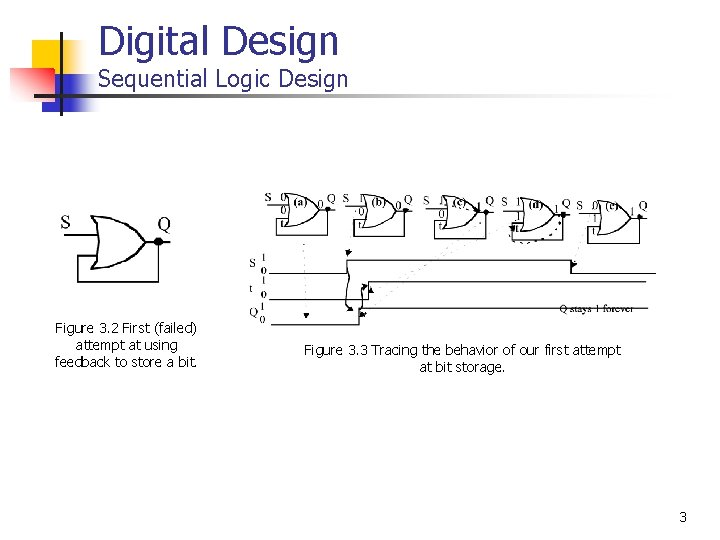Digital Design Sequential Logic Design Figure 3. 2 First (failed) attempt at using feedback to store a bit. Figure 3. 3 Tracing the behavior of our first attempt at bit storage. 3Digital Design Sequential Logic Design Figure 3. 5 SR latch when S=0 and R=1 Figure 3. 4 Basic SR Latch Figure 3. 6 SR latch when S=0 and R=0 4Digital Design Sequential Logic Design Figure 3. 7 SR latch when S=1 and R=0 Figure 3. 4 Basic SR Latch Figure 3. 8 SR latch when S=0 and R=0 5Digital Design Sequential Logic Design Figure 3. 9 Flight attendant call-button system using a basic SR latch. 6Digital Design Sequential Logic Design Figure 3. 10 S=1 and R=1 causes problems -- Q oscillates. Figure 3. 11 Q eventually settles to either 0 or 1, due to race condition. 7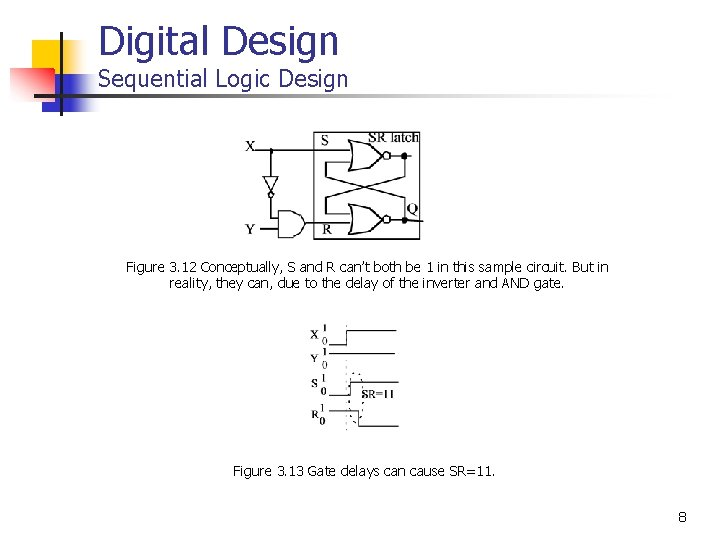Digital Design Sequential Logic Design Figure 3. 12 Conceptually, S and R can’t both be 1 in this sample circuit. But in reality, they can, due to the delay of the inverter and AND gate. Figure 3. 13 Gate delays can cause SR=11. 8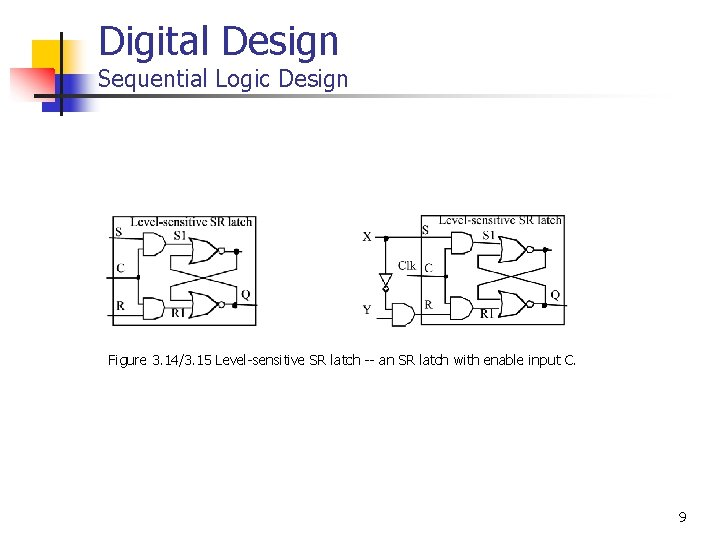Digital Design Sequential Logic Design Figure 3. 14/3. 15 Level-sensitive SR latch -- an SR latch with enable input C. 9Digital Design Sequential Logic Design Figure 3. 16 Symbol for dual-output level-sensitive SR latch. 10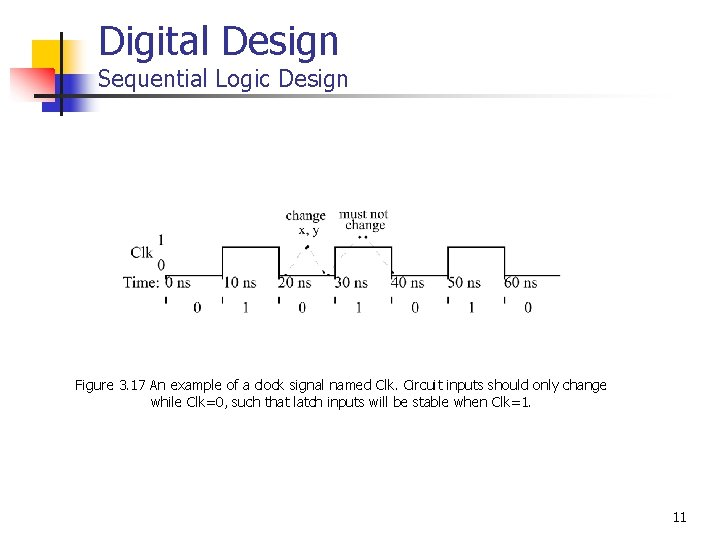Digital Design Sequential Logic Design Figure 3. 17 An example of a clock signal named Clk. Circuit inputs should only change while Clk=0, such that latch inputs will be stable when Clk=1. 11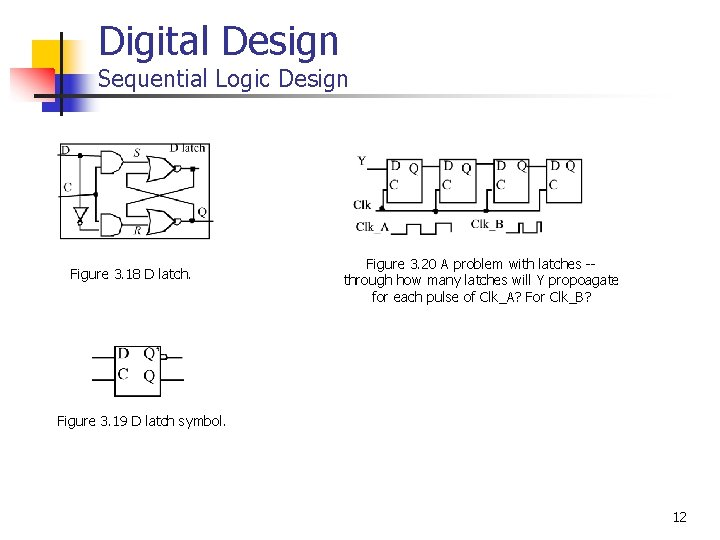Digital Design Sequential Logic Design Figure 3. 18 D latch. Figure 3. 20 A problem with latches -through how many latches will Y propoagate for each pulse of Clk_A? For Clk_B? Figure 3. 19 D latch symbol. 12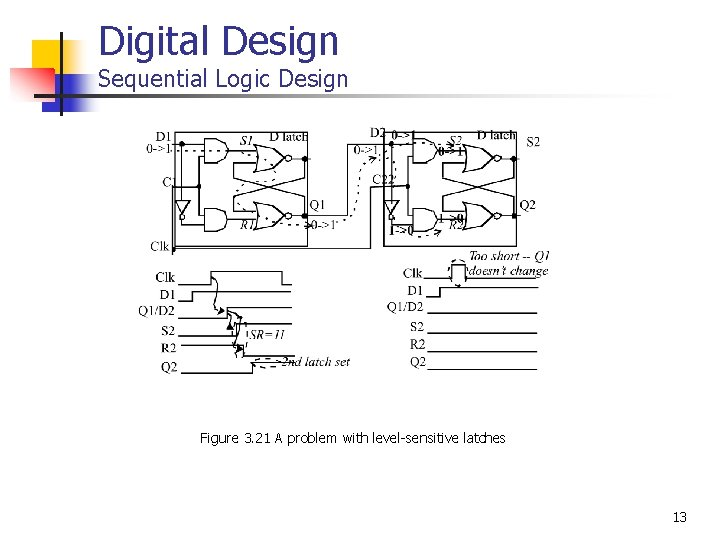Digital Design Sequential Logic Design Figure 3. 21 A problem with level-sensitive latches 13Digital Design Sequential Logic Design Figure 3. 23 A D flip-flop implementing an edge-triggered bit storage block, internally using two latches in a master-slave arrangement. 14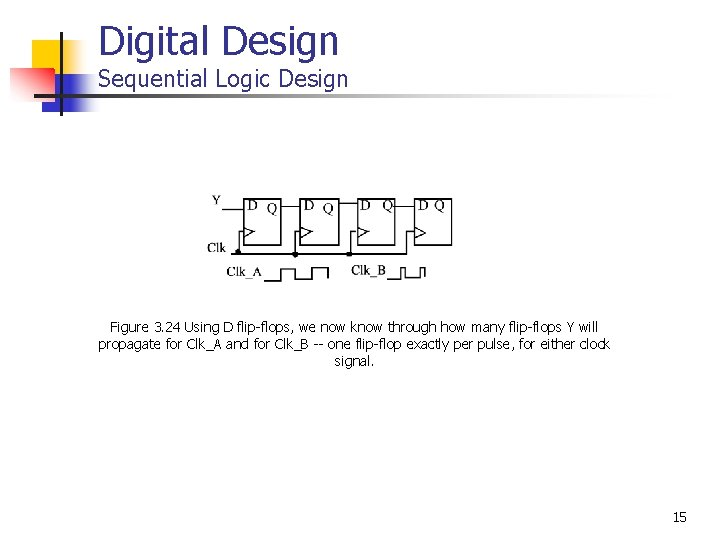Digital Design Sequential Logic Design Figure 3. 24 Using D flip-flops, we now know through how many flip-flops Y will propagate for Clk_A and for Clk_B -- one flip-flop exactly per pulse, for either clock signal. 15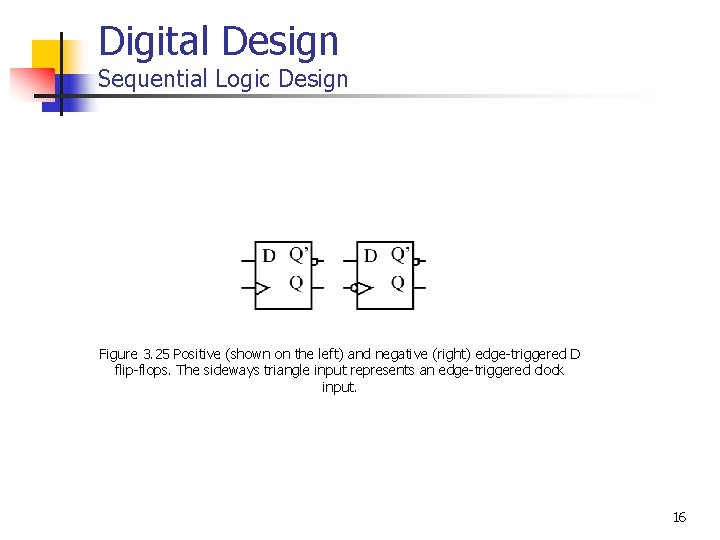Digital Design Sequential Logic Design Figure 3. 25 Positive (shown on the left) and negative (right) edge-triggered D flip-flops. The sideways triangle input represents an edge-triggered clock input. 16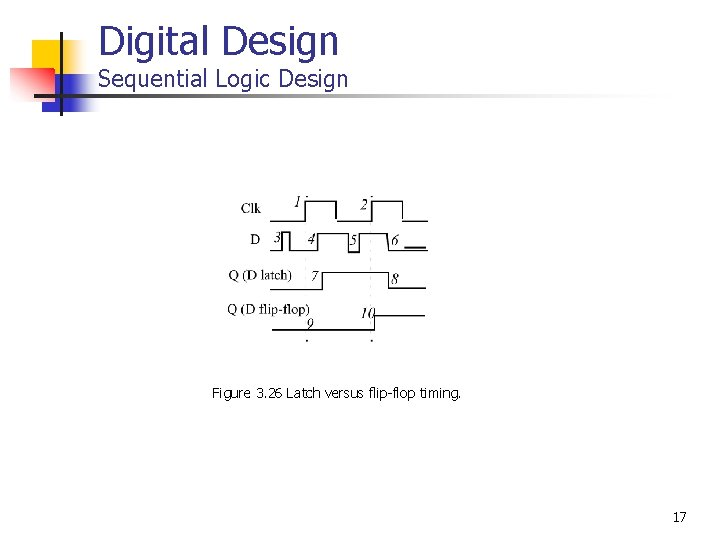Digital Design Sequential Logic Design Figure 3. 26 Latch versus flip-flop timing. 17Digital Design Sequential Logic Design Table 3. 1 D truth table for call-button system. Figure 3. 27 Flight attendant call-button system using a D flip-flop. 18Digital Design Sequential Logic Design S (set) SR latch S Level-sensitive. SR latch S 1 Q R (reset) Feature: S=1 sets Q to 1, R=1 resets Q to 0. Problem: SR=11 yield undefined Q. S D flip-flop D latch D C C R D Q Q R 1 Feature: S and R only have effect when C=1. We can design outside circuit so SR=11 never happens when C=1. Problem: avoiding SR=11 can be a burden. R Feature: SR can’t be 11 if D is stable before and while C=1, and will be 11 for only a brief glitch even if D changes while C=1. Problem: C=1 too long propagates new values through too many latches; too short may not enable a store. Clk D latch Dm Qm Cm master D latch Q’ Ds Qs’ Cs Qs Q slave Feature: Only loads D value present at rising clock edge, so values can’t propagate to other flip-flops during same clock cycle. Tradeoff: uses more gates internally than D latch, and requires more external gates than SR-but gate count is less of an issue today. Figure 3. 28 Increasingly-better bit storage blocks, leading to the D flip-flop. 19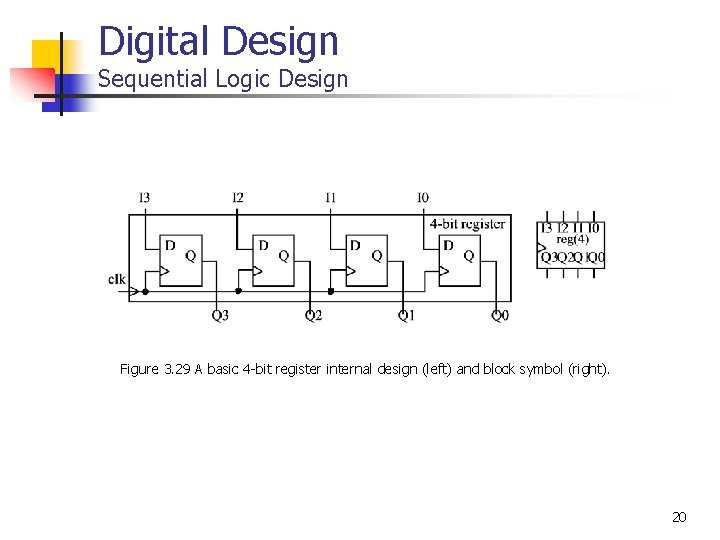Digital Design Sequential Logic Design Figure 3. 29 A basic 4 -bit register internal design (left) and block symbol (right). 20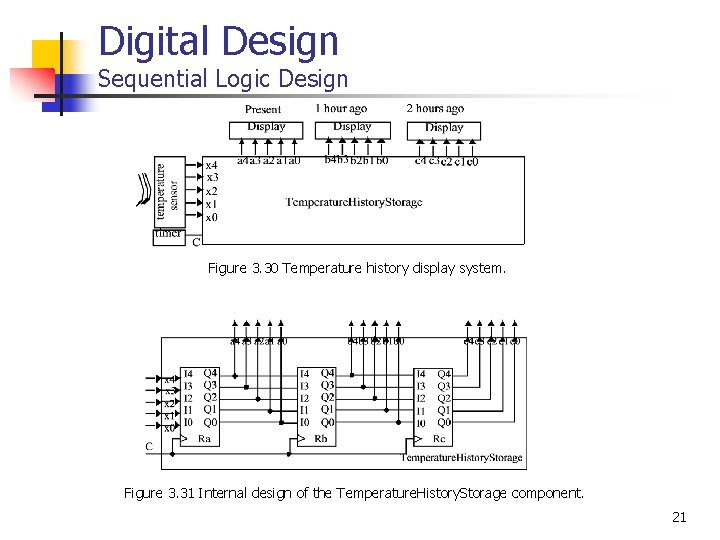Digital Design Sequential Logic Design Figure 3. 30 Temperature history display system. Figure 3. 31 Internal design of the Temperature. History. Storage component. 21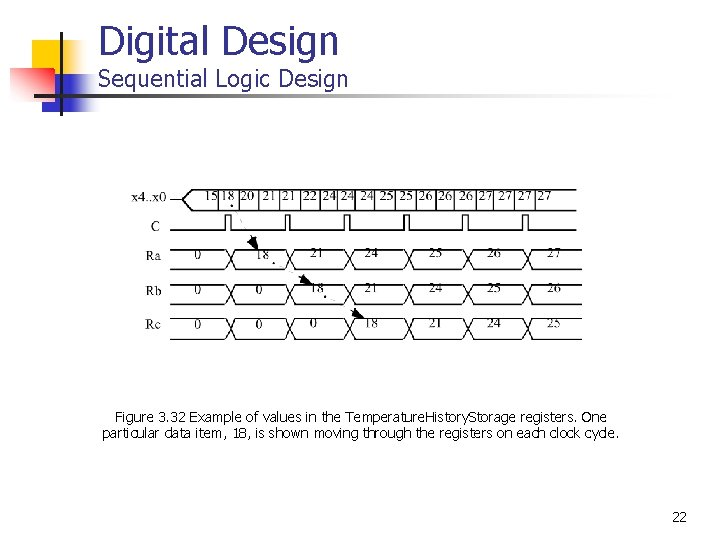Digital Design Sequential Logic Design Figure 3. 32 Example of values in the Temperature. History. Storage registers. One particular data item, 18, is shown moving through the registers on each clock cycle. 22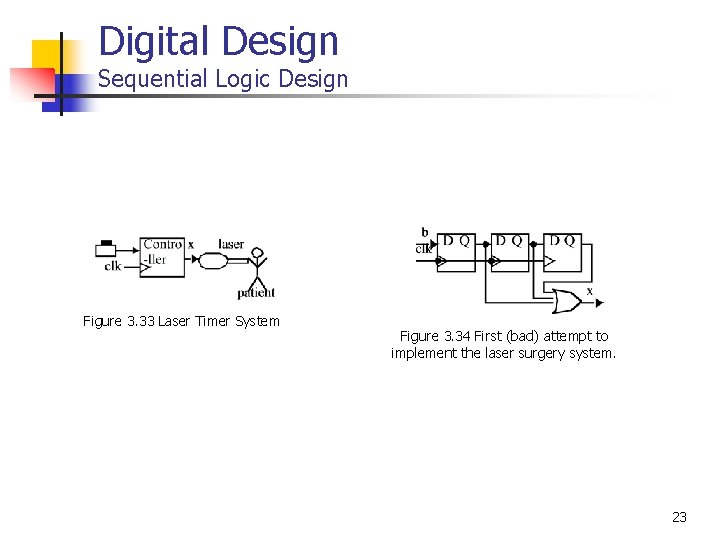Digital Design Sequential Logic Design Figure 3. 33 Laser Timer System Figure 3. 34 First (bad) attempt to implement the laser surgery system. 23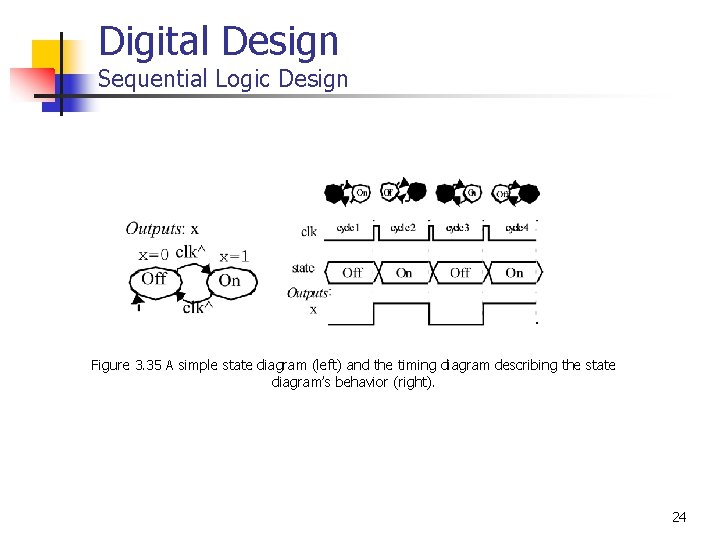Digital Design Sequential Logic Design Figure 3. 35 A simple state diagram (left) and the timing diagram describing the state diagram’s behavior (right). 24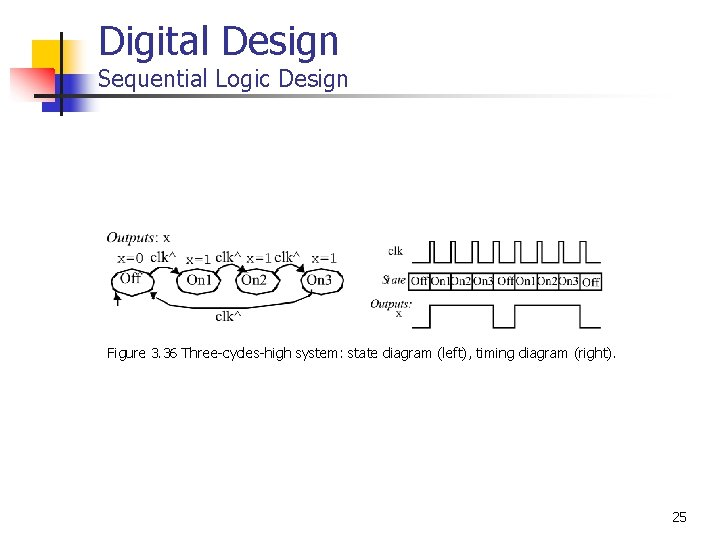Digital Design Sequential Logic Design Figure 3. 36 Three-cycles-high system: state diagram (left), timing diagram (right). 25Digital Design Sequential Logic Design Figure 3. 37 Three-cycles-high system: state diagram (left), timing diagram (right). 26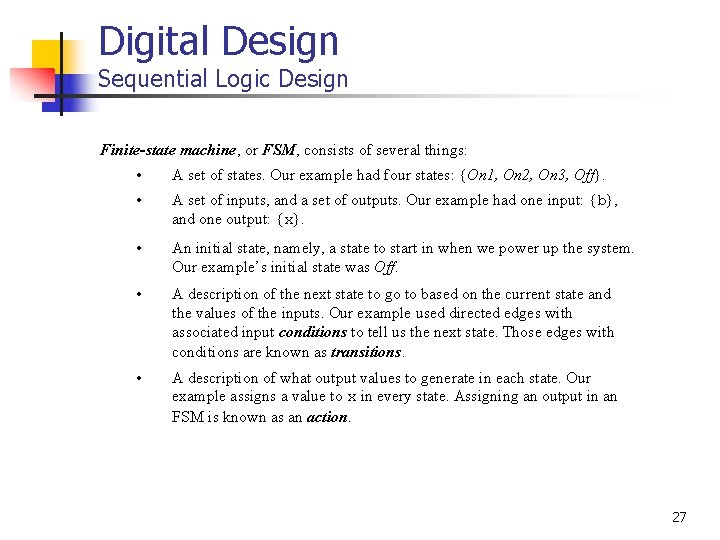Digital Design Sequential Logic Design Finite-state machine, or FSM, consists of several things: • A set of states. Our example had four states: {On 1, On 2, On 3, Off}. • A set of inputs, and a set of outputs. Our example had one input: {b}, and one output: {x}. • An initial state, namely, a state to start in when we power up the system. Our example’s initial state was Off. • A description of the next state to go to based on the current state and the values of the inputs. Our example used directed edges with associated input conditions to tell us the next state. Those edges with conditions are known as transitions. • A description of what output values to generate in each state. Our example assigns a value to x in every state. Assigning an output in an FSM is known as an action. 27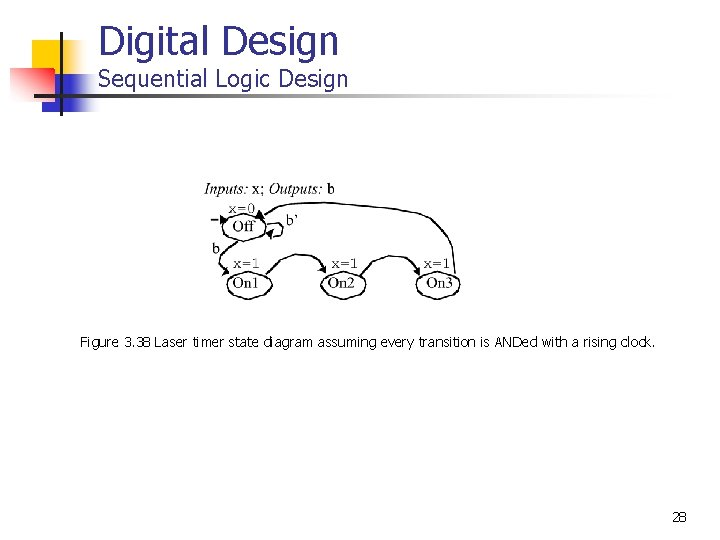Digital Design Sequential Logic Design Figure 3. 38 Laser timer state diagram assuming every transition is ANDed with a rising clock. 28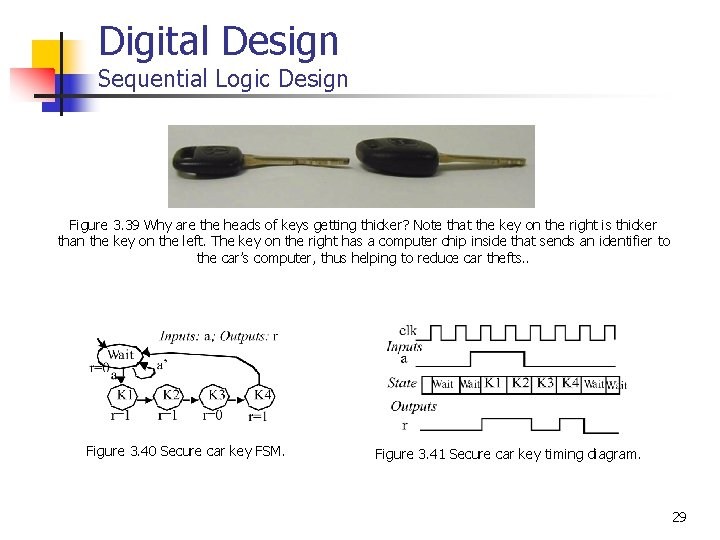Digital Design Sequential Logic Design Figure 3. 39 Why are the heads of keys getting thicker? Note that the key on the right is thicker than the key on the left. The key on the right has a computer chip inside that sends an identifier to the car’s computer, thus helping to reduce car thefts. . Figure 3. 40 Secure car key FSM. Figure 3. 41 Secure car key timing diagram. 29Digital Design Sequential Logic Design Figure 3. 44 Code detector FSM. 30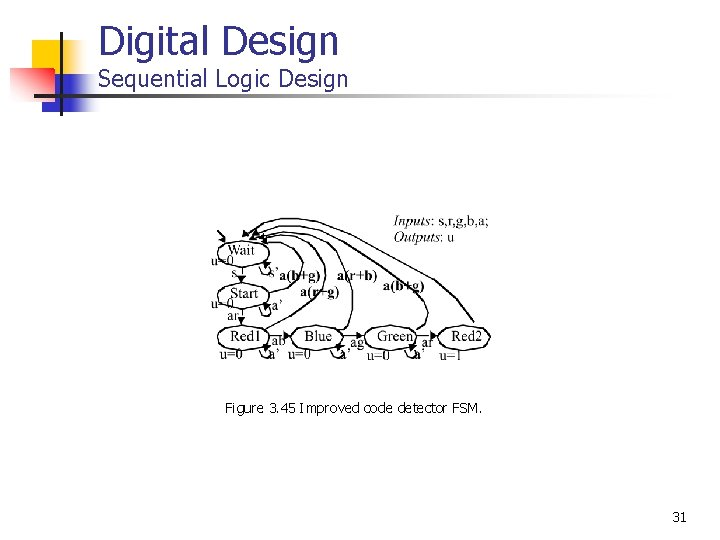Digital Design Sequential Logic Design Figure 3. 45 Improved code detector FSM. 31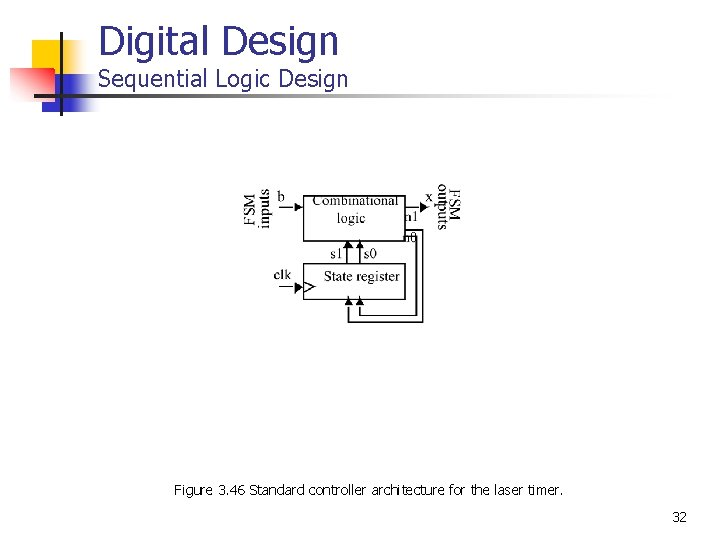Digital Design Sequential Logic Design Figure 3. 46 Standard controller architecture for the laser timer. 32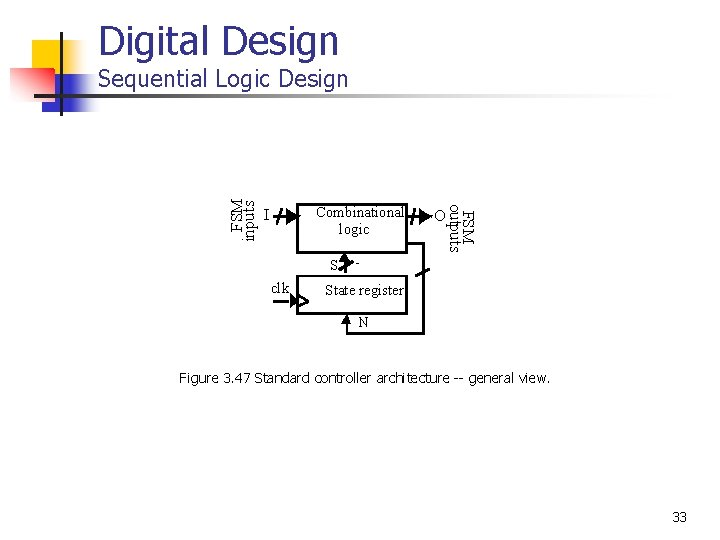Digital Design Combinational logic I FSM outputs FSM inputs Sequential Logic Design O S clk State register N Figure 3. 47 Standard controller architecture -- general view. 33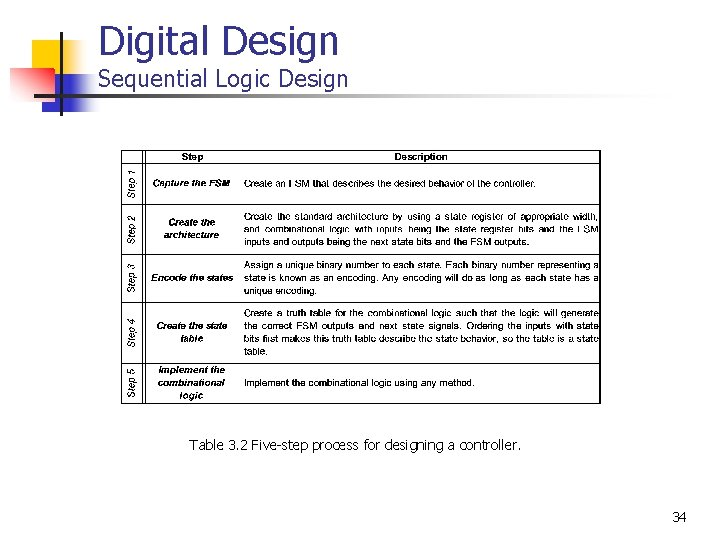Digital Design Sequential Logic Design Table 3. 2 Five-step process for designing a controller. 34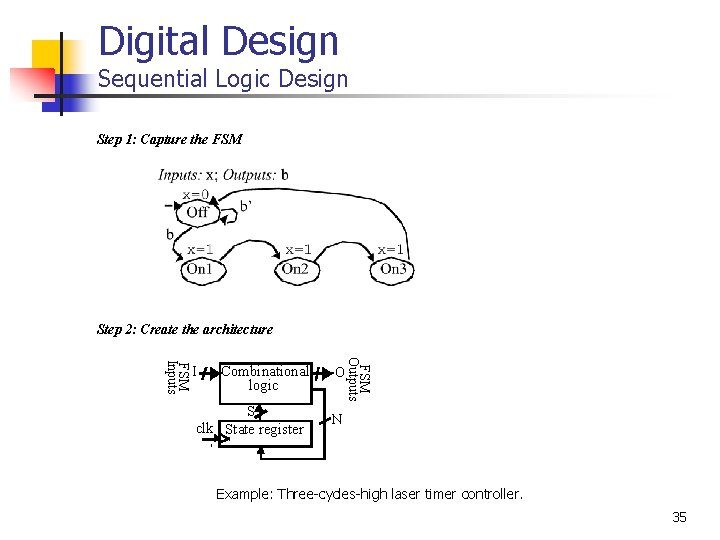Digital Design Sequential Logic Design Step 1: Capture the FSM Step 2: Create the architecture Combinational logic S clk State register O FSM Outputs FSM Inputs I N Example: Three-cycles-high laser timer controller. 35Digital Design Sequential Logic Design Step 3: Encode the states Step 4: Create the state table Example: Three-cycles-high laser timer controller. 36Digital Design Sequential Logic Design Step 5: Implement the combinational logic Example: Three-cycles-high laser timer controller. 37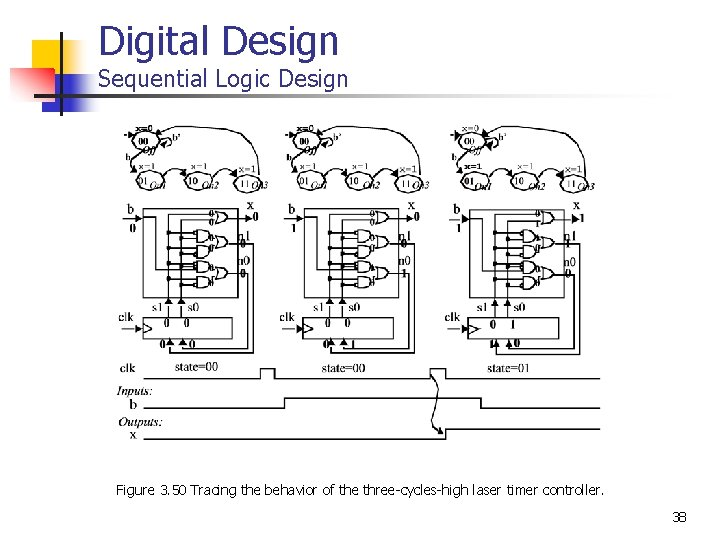Digital Design Sequential Logic Design Figure 3. 50 Tracing the behavior of the three-cycles-high laser timer controller. 38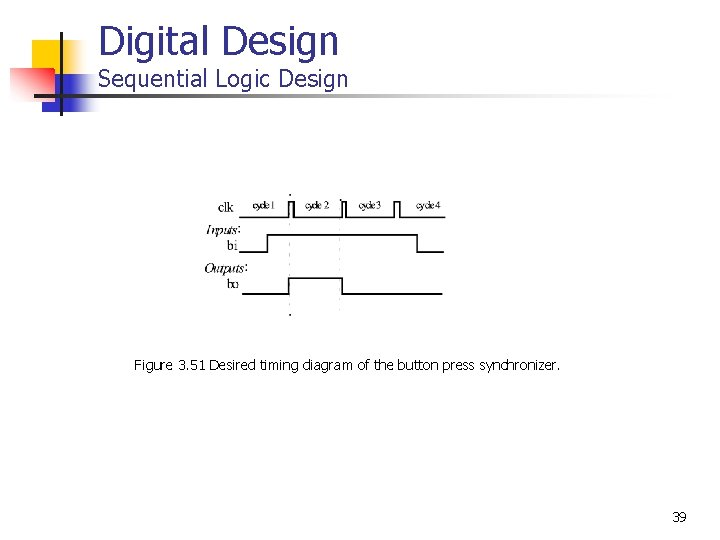Digital Design Sequential Logic Design Figure 3. 51 Desired timing diagram of the button press synchronizer. 39Digital Design Sequential Logic Design Figure 3. 52 Button press synchronizer design steps: (a) initial FSM, (b) architecture, (c) FSM with encoded states, (d) state table, (e) final circuit with implemented combinational logic. 40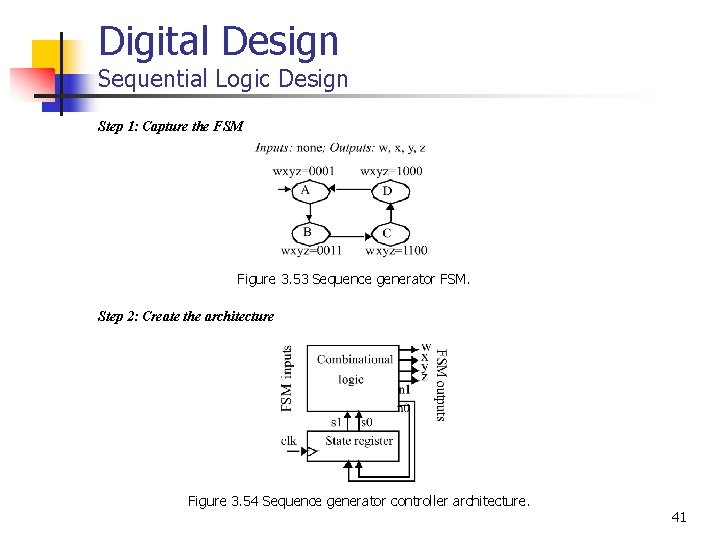Digital Design Sequential Logic Design Step 1: Capture the FSM Figure 3. 53 Sequence generator FSM. Step 2: Create the architecture Figure 3. 54 Sequence generator controller architecture. 41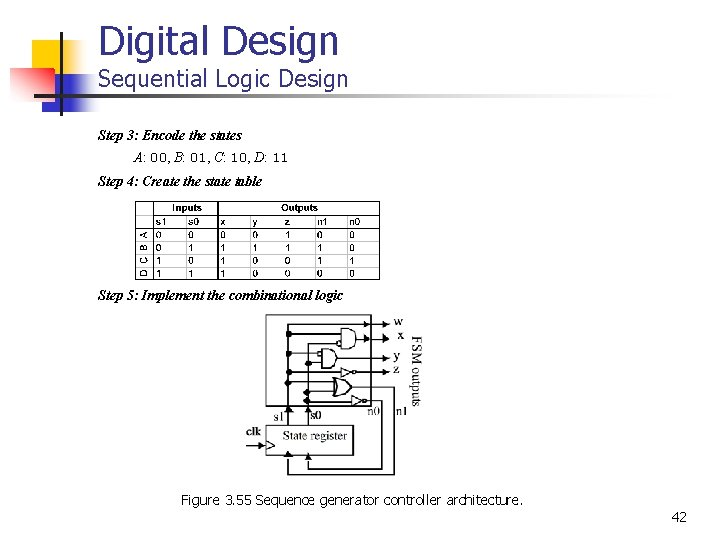Digital Design Sequential Logic Design Step 3: Encode the states A: 00, B: 01, C: 10, D: 11 Step 4: Create the state table Step 5: Implement the combinational logic Figure 3. 55 Sequence generator controller architecture. 42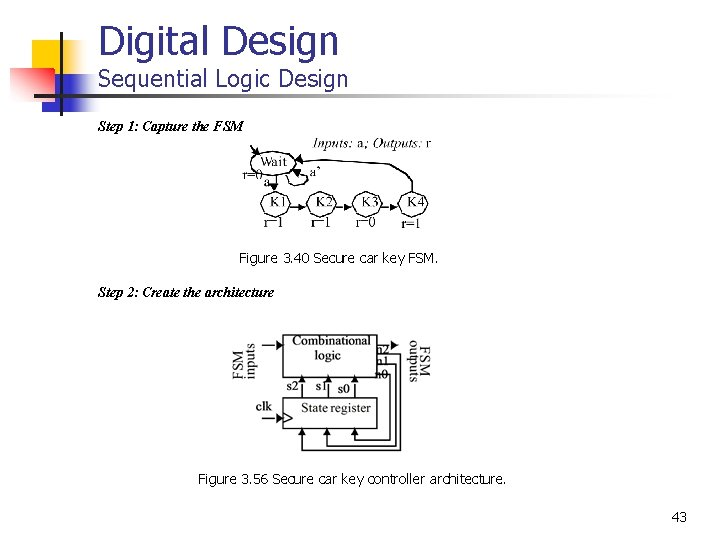Digital Design Sequential Logic Design Step 1: Capture the FSM Figure 3. 40 Secure car key FSM. Step 2: Create the architecture Figure 3. 56 Secure car key controller architecture. 43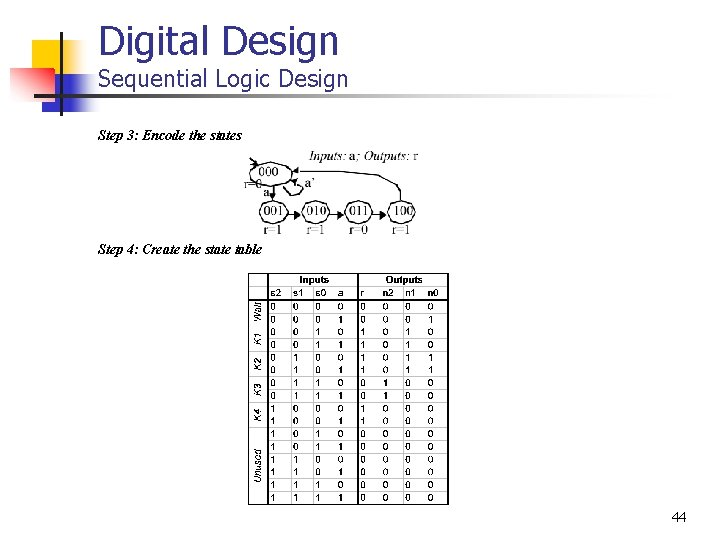Digital Design Sequential Logic Design Step 3: Encode the states Step 4: Create the state table 44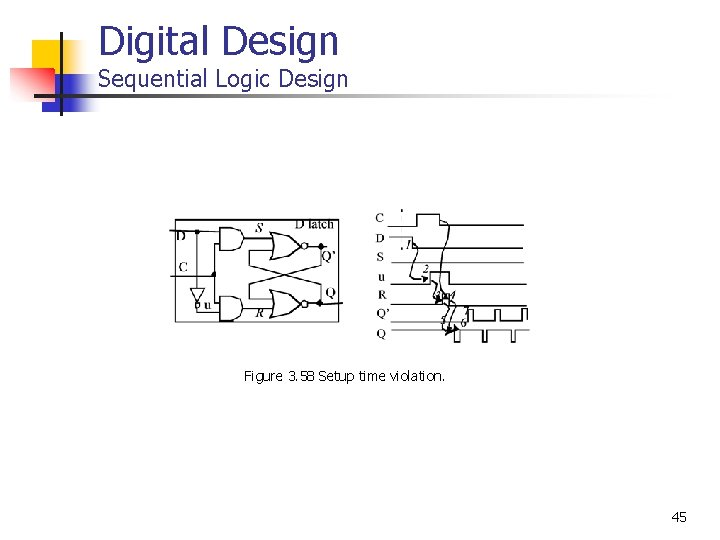Digital Design Sequential Logic Design Figure 3. 58 Setup time violation. 45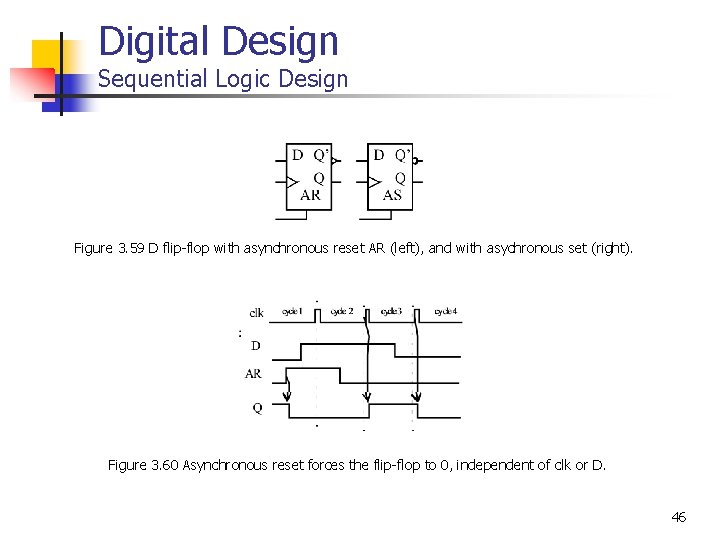Digital Design Sequential Logic Design Figure 3. 59 D flip-flop with asynchronous reset AR (left), and with asychronous set (right). Figure 3. 60 Asynchronous reset forces the flip-flop to 0, independent of clk or D. 46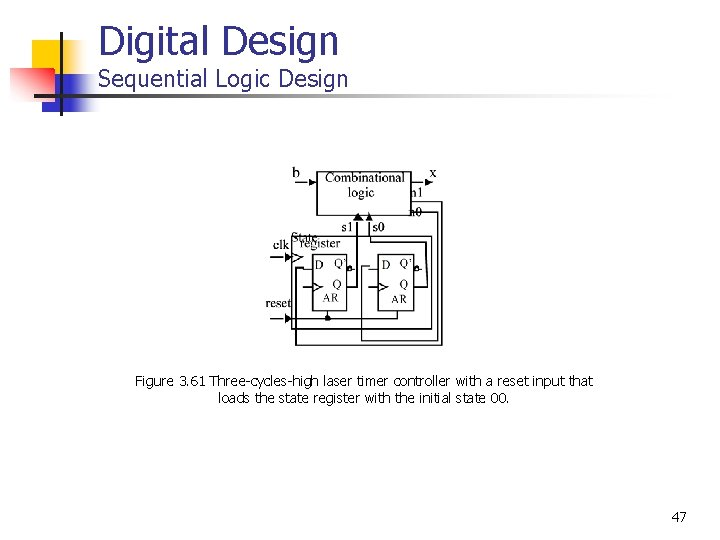Digital Design Sequential Logic Design Figure 3. 61 Three-cycles-high laser timer controller with a reset input that loads the state register with the initial state 00. 47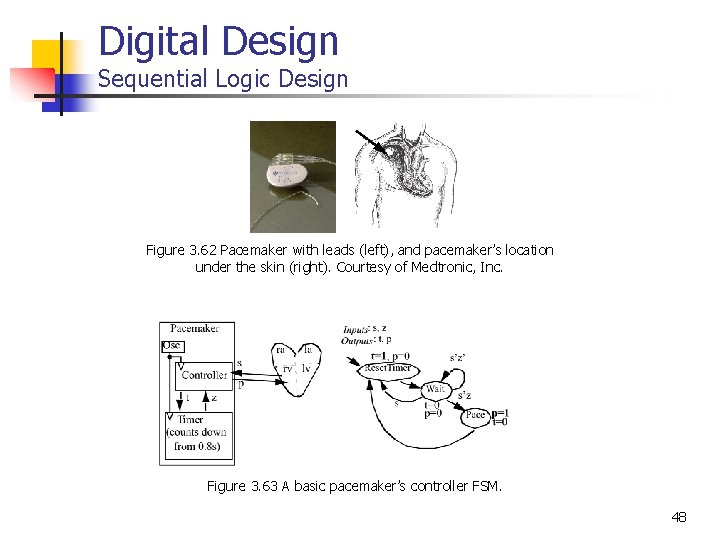Digital Design Sequential Logic Design Figure 3. 62 Pacemaker with leads (left), and pacemaker’s location under the skin (right). Courtesy of Medtronic, Inc. Figure 3. 63 A basic pacemaker’s controller FSM. 48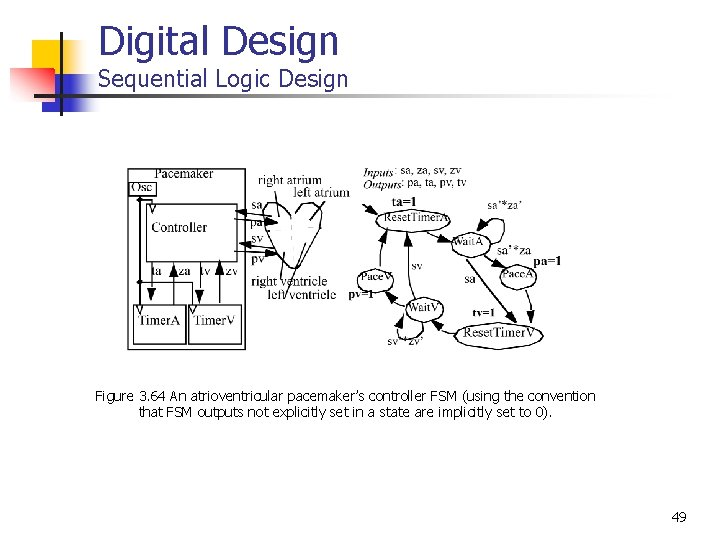Digital Design Sequential Logic Design Figure 3. 64 An atrioventricular pacemaker’s controller FSM (using the convention that FSM outputs not explicitly set in a state are implicitly set to 0). 49